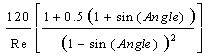Close

The head loss hL can be calculated as:

hL = kloss x (um2 / (2 x g) )

Where kloss is the head loss coefficient, um is the flow velocity in the pipe, and g is the gravitational acceleration. kloss is dependant on the Reynolds Number Re. If Re is smaller than or equal to 50, then (1 - 50 / Re) is taken to be zero:

kloss =+ (1 - 50 / Re) kqu

Where kqu is a function of the angle and the number of  louvers based on a table of values (Ref), and Re is the Reynolds Number and is calculated as:

Re = um x Dh / v

Where v is the kinematic viscosity of air and Dh is the hydraulic diameter of the tube. A tube of square cross section has a hydraulic diameter equal to the width of the tube.

References

Idel'chik, I E. and Fried, Erwin., Flow Resistance, a Design Guide for Engineers, Taylor & Francis, Washington D.C. 1989. p 306.